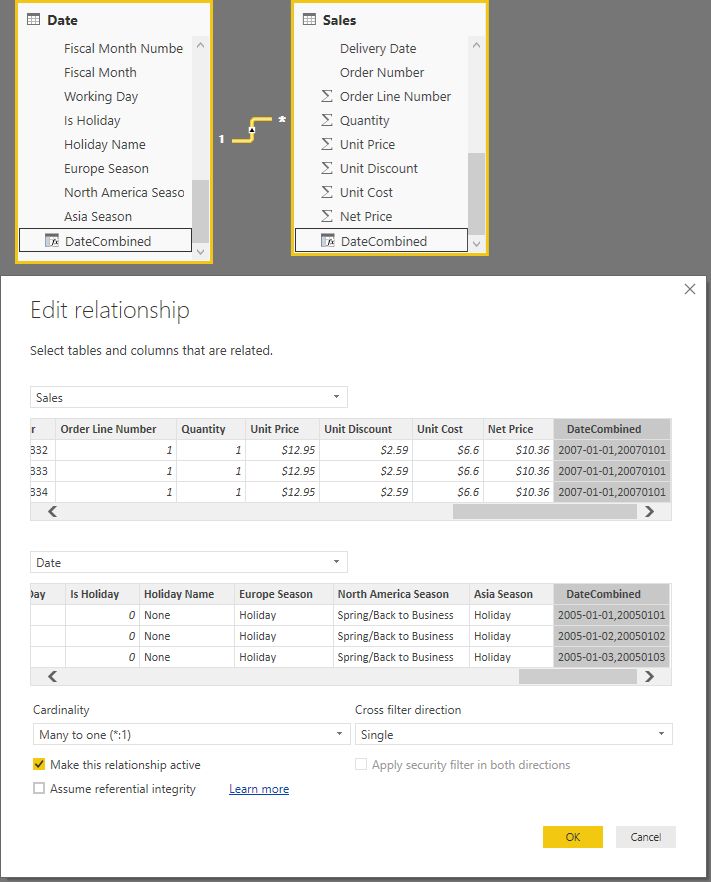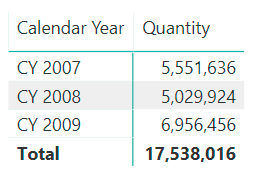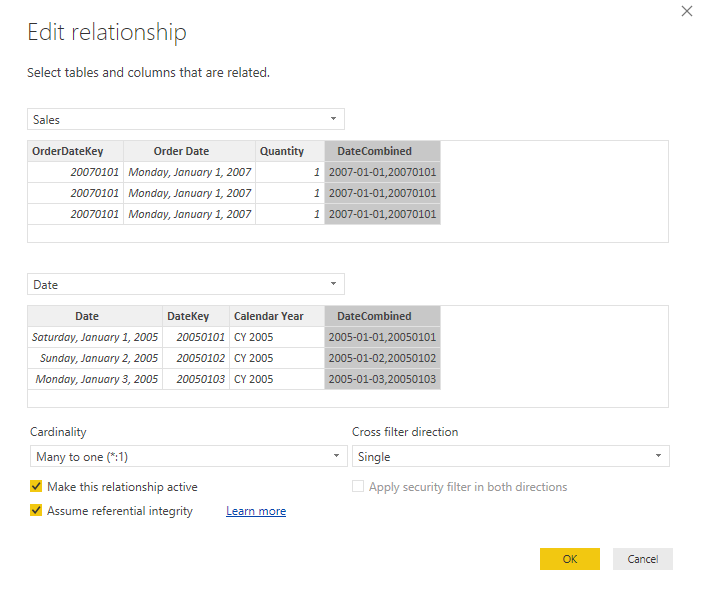The DAX language manages data models in Power BI, Power Pivot, and Analysis Services Tabular. To simplify the reference to data models where DAX can be used, we call them Tabular models. A relationship in a Tabular model connects two tables only using one column as an identifier. While this seems like a limitation, it reflects the behavior of the internal VertiPaq engine, which is an in-memory columnar database.

## Managing relationships based on multiple columns

When you import data in a Tabular model, relationships are optimized when they are based on a single column that does not have a high number of unique values. Columns that do have a high number of unique values are known as high cardinality columns. If two tables require two or more columns to define a relationship, it is possible to create a calculated column that concatenates the values of the columns used for the relationship, on both tables. This way, the relationship is based on one single column on each side. However, the resulting calculated column will have a higher cardinality than the original columns, which is not good for performance.

Most of the time, the presence of relationships based on multiple columns suggests that a better denormalization of the model is required to obtain an optimal star schema. Nevertheless, for smaller tables or when it is not possible to apply complex transformations, concatenating columns is the preferred way to obtain the relationships required. When this happens with data loaded in memory, the only concern is the cardinality of the resulting column. However, this is not the main concern in DirectQuery mode.

A calculated column in DirectQuery translates its expression into a native SQL expression. When this is used as a JOIN condition to express the relationship in a Tabular model, this could produce a non-optimal query plan, which could introduce unnecessary slowness in the query execution. For this reason, Microsoft introduced COMBINEVALUES, which is a function expressly designed to optimize relationships based on multiple columns in DirectQuery mode.

## Concatenating columns as strings

We created a simulation of the scenario by enforcing a relationship between Sales and Date using two columns: Order Date (a date) and OrderDateKey (an integer). The relationship uses two calculated columns named DateCombined, defined as follows:

```'Date'[DateCombined] = 'Date'[Date] &  "," & 'Date'[DateKey]
Sales[DateCombined] = Sales[Order Date] & "," & Sales[OrderDateKey]
```

The relationship in the model uses the DateCombined column.The data type of the DateCombined column is a string.

By creating a simple matrix showing the sum of quantity by calendar year, one obtains the following report.The underlying DAX code generated for the visual is the following.

```EVALUATE
TOPN (
502,
SUMMARIZECOLUMNS (
ROLLUPADDISSUBTOTAL ( 'Date'[Calendar Year], "IsGrandTotalRowTotal" ),
"SumQuantity", CALCULATE ( SUM ( 'Sales'[Quantity] ) )
),
[IsGrandTotalRowTotal], 0,
'Date'[Calendar Year], 1
)
ORDER BY
[IsGrandTotalRowTotal] DESC,
'Date'[Calendar Year]
```

DirectQuery produces a single SQL query with the following structure.

```SELECT
TOP (1000001) [t0].[Calendar Year],
SUM(CAST([t1].[Quantity] as BIGINT)) AS [a0]
FROM
[t1]
LEFT OUTER JOIN [t0]
ON ([t1].[DateCombined] = [t0].[DateCombined])
GROUP BY
[t0].[Calendar Year]
```

The JOIN condition in the syntax above is very expensive. Indeed, it combines the result of two expressions that dynamically create a string for each row of Sales and Date, as you can see in the following definitions of t0 and t1. This approach is usually not optimized, because it is hard for the engine to use existing indexes to optimize the query plan.

The t0 alias comes from the Date table:

```SELECT
[t0].[Date] AS [Date],
[t0].[DateKey] AS [DateKey],
[t0].[Calendar Year] AS [Calendar Year],
(
COALESCE(CAST([t0].[Date] AS VARCHAR(4000)), '') + (
N',' + COALESCE(CAST([t0].[DateKey] AS VARCHAR(4000)), '')
)
) AS [DateCombined]
FROM
(
SELECT
[Date],
[DateKey],
[Calendar Year]
FROM
[Analytics].[Date] as [\$Table]
) AS [t0]
```

The t1 alias comes from the Sales table:

```SELECT
[t1].[OrderDateKey] AS [OrderDateKey],
[t1].[Order Date] AS [Order Date],
[t1].[Quantity] AS [Quantity],(
COALESCE(CAST([t1].[Order Date] AS VARCHAR(4000)), '') + (
N',' + COALESCE(CAST([t1].[OrderDateKey] AS VARCHAR(4000)), '')
)
) AS [DateCombined]
FROM
(
(
SELECT
[OrderDateKey],
[Order Date],
[Quantity]
FROM
[Analytics].[Sales - Complete] as [\$Table]
)
) AS [t1]
```

## Using COMBINEVALUES

You get the same result using COMBINEVALUES as you did using the string concatenation seen previously. However, the syntax of this function provides more information to DirectQuery about the underlying columns. DirectQuery can in turn, optimize the resulting SQL code.

We changed the calculated columns definition using the syntax below.

```'Date'[DateCombined] = COMBINEVALUES ( ",", 'Date'[Date], 'Date'[DateKey] )
Sales[DateCombined] = COMBINEVALUES ( ",", Sales[Order Date], Sales[OrderDateKey] )
```

This way, the same DAX query we have seen in the previous example generates the following SQL code:

```SELECT
TOP (1000001) [t0].[Calendar Year],
SUM(CAST([t1].[Quantity] as BIGINT)) AS [a0]
FROM
[t1]
LEFT OUTER JOIN [t0]
ON (
(
[t1].[Order Date] = [t0].[Date]
OR [t1].[Order Date] IS NULL
AND [t0].[Date] IS NULL
)
AND (
[t1].[OrderDateKey] = [t0].[DateKey]
OR [t1].[OrderDateKey] IS NULL
AND [t0].[DateKey] IS NULL
)
)
GROUP BY
[t0].[Calendar Year]
```

Please note that t0 and t1 have the same definition as seen in the previous example. Thus, they both contain the definition of the DateCombined column, which is never used in the query. For this reason, the query plan optimizes the execution, and the JOIN can partially leverage existing indexes.

At this point, further optimization can be achieved by enabling the “Assume referential integrity” option in the relationship between Sales and Date in the Power BI model.This way, the SQL code generated by DirectQuery will use an INNER JOIN instead of a LEFT JOIN. However, this will not remove the need for the OR condition that checks for the existence of a NULL value in the two columns.

Finally, the DirectQuery engine should optimize the SQL code in case the columns used in COMBINEVALUES are marked as not nullable (NOT NULL) in the relational engine. However, this does not happen with the current version of Power BI (April 2018), but once the column state is correctly identified by a future version of Power BI or Analysis Services (maybe the July 2018 version, or close to that time), the expected code should be the following:

```SELECT
TOP (1000001) [t0].[Calendar Year],
SUM(CAST([t1].[Quantity] as BIGINT)) AS [a0]
FROM
[t1]
LEFT OUTER JOIN [t0]  -- or INNER JOIN here if Assume referential integrity is on
ON
[t1].[Order Date] = [t0].[Date]
AND [t1].[OrderDateKey] = [t0].[DateKey]
GROUP BY
[t0].[Calendar Year]
```

## Conclusions

Relationships based on multiple columns should be avoided in a Tabular model – even in DirectQuery. The relationships based on a single native column always produce a better query plan in DirectQuery. When a native column is not available, then COMBINEVALUES should be used to create a calculated column in two tables to define a relationship between them. The SQL code is not optimal yet, but it usually produces a much better query execution plan than other expressions that would combine the values of multiple columns into a single column.

COMBINEVALUESCombines the given set of operands using a specified delimiter.

`COMBINEVALUES ( <Delimiter>, <Expression1>, <Expression2> [, <Expression2> [, … ] ] )`

LEFTReturns the specified number of characters from the start of a text string.

`LEFT ( <Text> [, <NumberOfCharacters>] )`

ORReturns TRUE if any of the arguments are TRUE, and returns FALSE if all arguments are FALSE.

`OR ( <Logical1>, <Logical2> )`